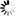» Featured Products » CNC Spindles & VFDs » VFDs » KL-VFD05 Mini-type Integrated Universal Inverter (VFD), 2.2KW

Product in stock

## Price

\$149.95

### KL-VFD05 Mini-type Integrated Universal Inverter (VFD), 2.2KW

#### Product Description

Model Number: E500-2S0022

Input: 220VAC ±15%  1 or 3 Phase (R,S or R, T or S,T)    50/60Hz

Output: 220VAC 3 Phase (U,V,W)   2.2kw

Output Current:10A

Output Frequency: 0-400HZ

Type: Other

Rated capacity:3.8KVA

Rated output current:10A

Control Mode: V/F control

Running: low noise running

F0.00 = 1 (or 3 if you want to test using the knob on the VFD)

F0.02=1001 (or 1000 if you want to test using the knob on VFD)

F0.04= 400 or 300 set according your spindle
F0.05=10
F0.06=10
F0.11=0

F0.12=400 or 300 set according your spindle

F0.13=220

F1.00 =0

F1.01 =10V

F1.03=0

F1.04 =400

F3.08=3 (Show spindle speed on screen)

F2.19=1 ( If your spindle is 2 pole)

F1.08=11 ( Terminal X1 as a Run)

F1.14=8 ( Inverter Fault signal Output)

TA, Tc connect to fault output

X1 and Com connect to Run (or relay)

AI1 and GND ( 0-10VDC)

one year manufacture warranty

For 0-10V DC : Connect to AI and GND

there is no RS485 included in this VFD

1. Press [SET]
2. Use arrow keys to change to program number your looking to change
3. Press [SET]
4. Use the arrow keys to change the value to appropriate value
5. Press [SET] to save the value
6. Press [ESC] to exit programming mode

### Setting :

• F0.0 = 1 (for 0-10V Control) or 3 (for knob control)
• F0.2 = 1001 (for panel [RUN] button)
• F0.4= 400 (Spindle Frequency)
• F0.5 = 5.0 (Acceleration time)
• F0.11 = 0 (Torque Boost)
• F0.12 = 400 (Basic Frequency)
• F0.13 = 220 (Volts)
• F1.00 = 0.4 (AI Lower Limit)
• F1.01 = 10 (AI Upper Limit)
• F1.03 = 0 (Min Freq)
• F1.04 = 400 (Max Freq)
• F1.08 = 11 (Terminal 1 Function)Updating...